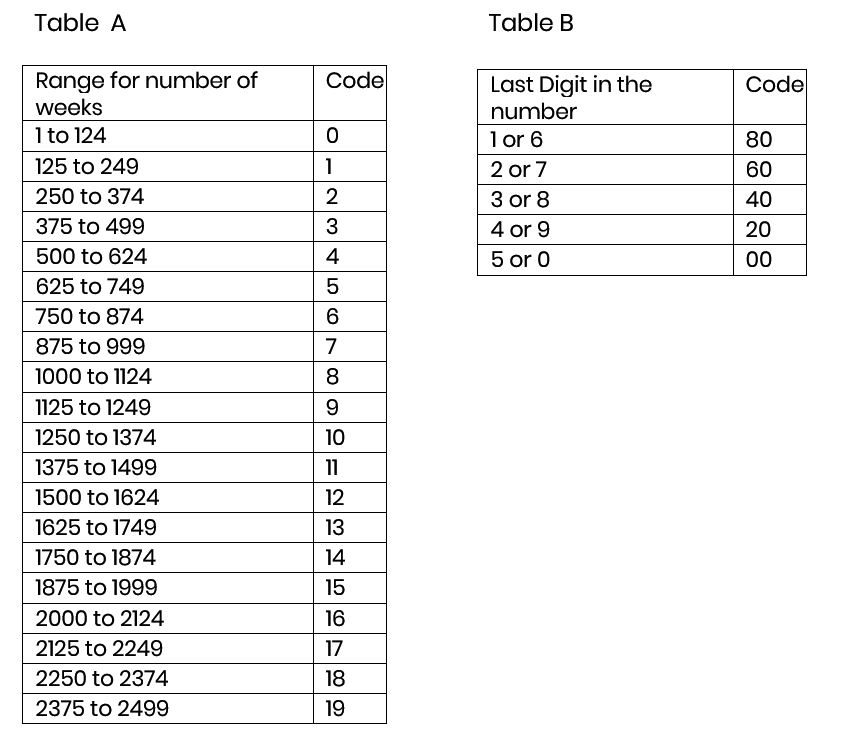April 2023

# Convert Weeks into Minutes

0
0

This is a very simple arithmetic problem .Just multiply number of weeks by 7*24*60 =10080 and you get the result. On the stage can we do such multiplications without errors and right the results from right to left (most significant digit first)? Here is an approach which requires two tables.Example : Find the number of minutes in 1439 weeks. Answer = 1439*10080 = 14505120 minutes

Faster Method :

Step 1: From Table A we get 11 as the code. Add 11 to 1439 to get 1450.

These are the first 4 digits of the answer. Step 2: Round off the last digit to 5 or 0.

The resulting number is 1440.Take 3 last digits(440). Divide it by 5 to get 88. Subtract 88 to 1439 is 1351 and retain last two digits. We get 51.

They form next two numbers of the result.

Step 3: From Table B, find the code for last digit of 1439. The code is 20.

They form the last 2 digits and the answer is 14505120

Explanation of the Method:

Note that finding number of minutes in a week is the problem of multiplying number of weeks by the number 10080. Therefore, if the number of weeks is a 4 digit number, say 1567, we can multiply it with 10080 as follows.

1567 x 10080 =

15670000 +

125360 = 15795360.

By Mr. Subramani. K, Staff Member, IIIT Bangalore
Email : subramani.k@iiitb.ac.inThe International Institute of Information Technology Bangalore is a technical and research university in Bangalore, India. The Institute is a registered not-for-profit society funded jointly by the Government of Karnataka and the IT industry under a public-private partnership model.

For more details: Visit www.iiitb.ac.in

Featured Stories